Question

The stoichiometric relationship between the reactants and products of an aqueous reaction can be used to determine different kinds of information about a reaction, such as the volume of a given molarity reactant required to produce a certain amount of product.

Hydrochloric acid (HCl) reacts with sodium carbonate (Na2CO3), forming sodium chloride (NaCl), water (H2O), and carbon dioxide (CO2). This equation is balanced as written:

2HCl(aq)+Na2CO3(aq)→2NaCl(aq)+H2O(l)+CO2(g)

a. What volume of 2.50 M HCl in liters is needed to react completely (with nothing left over) with 0.250 L of 0.300 M Na2CO3?

Express your answer to three significant figures and include the appropriate units.

b.A 317-mL sample of unknown HCl solution reacts completely with Na2CO3 to form 13.1 g CO2. What was the concentration of the HCl solution?

Express your answer to three significant figures and include the appropriate units.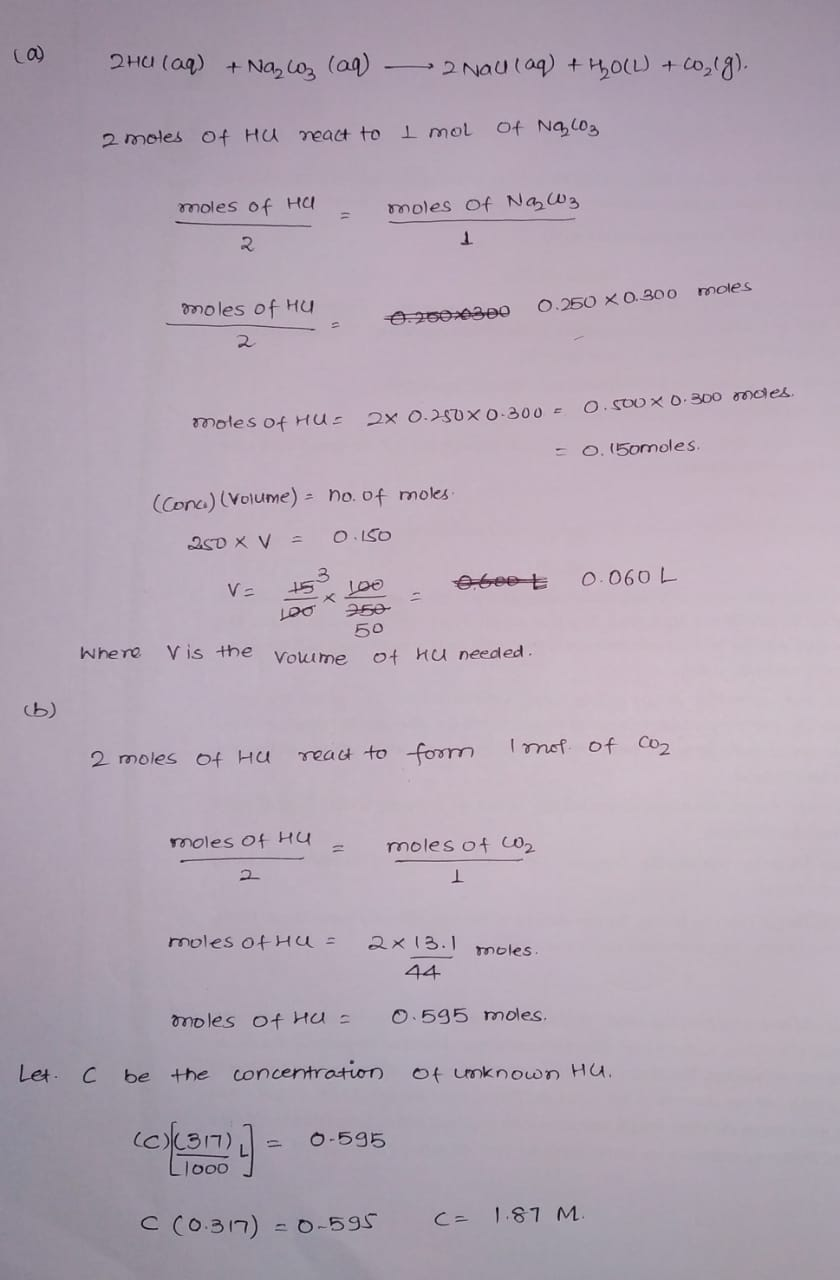there was a slight calculation error in the previous answer. i have corrected and uploaded it again. please check it! thanks

#### Earn Coins

Coins can be redeemed for fabulous gifts.

Similar Homework Help Questions
• ### 4. The stoichiometric relationship between the reactants and products of an aqueous reaction can be used...

4. The stoichiometric relationship between the reactants and products of an aqueous reaction can be used to determine different kinds of information about a reaction, such as the volume of a given molarity reactant required to produce a certain amount of product. Hydrochloric acid (HCl) reacts with sodium carbonate (Na2CO3), forming sodium chloride (NaCl), water (H2O), and carbon dioxide (CO2). This equation is balanced as written: 2HCl(aq)+Na2CO3(aq)→2NaCl(aq)+H2O(l)+CO2(g) A.) What volume of 3.00 M HCl in liters is needed to react...

• ### The stoichiometric relationship between the reactants and products of an aqueous reaction can be used to...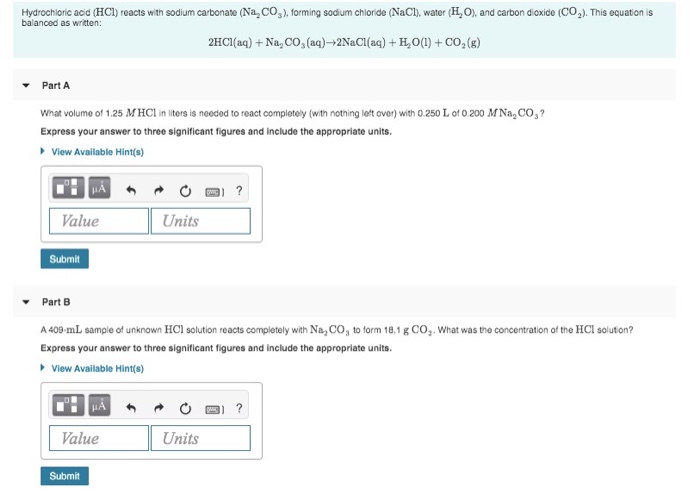The stoichiometric relationship between the reactants and products of an aqueous reaction can be used to determine different kinds of information about a reaction, such as the volume of a given molarity reactant required to produce a certain amount of product. Hydrochloric acid (HCl) reacts with sodium carbonate (Na CO2). forming sodium chloride (NaCl), water (HO), and carbon dioxide (C0.). This equation is balanced as written: 2HCl(aq) + Na, CO, (aq)-2NaCl(aq) + H,0(1) + CO2(g) Part A What volume of...

• ### 1. What volume of 1.00 M HCl in liters is needed to react completely (with nothing...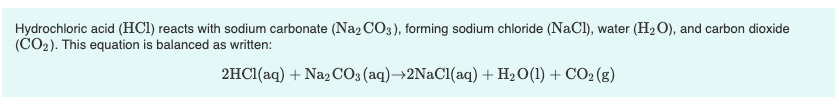1. What volume of 1.00 M HCl in liters is needed to react completely (with nothing left over) with 0.750 L of 0.200 M Na2CO3? Express your answer to three significant figures and include the appropriate units. 2. A 639-mL sample of unknown HCl solution reacts completely with Na2CO3 to form 15.1 g CO2. What was the concentration of the HCl solution? Express your answer to three significant figures and include the appropriate units. Hydrochloric acid (HCl) reacts with sodium...

• ### Solution Stoichiometry Hydrochloric acid (HCl) reacts with sodium carbonate (Na2CO3), forming sodium chloride (NaCl), water (H2O),...

Solution Stoichiometry Hydrochloric acid (HCl) reacts with sodium carbonate (Na2CO3), forming sodium chloride (NaCl), water (H2O), and carbon dioxide (CO2). This equation is balanced as written: 2HCl(aq)+Na2CO3(aq)→2NaCl(aq)+H2O(l)+CO2(g) a) What volume of 2.75 M HCl in liters is needed to react completely (with nothing left over) with 0.750 L of 0.300 M Na2CO3? b) A 565-mL sample of unknown HCl solution reacts completely with Na2CO3 to form 10.1 g CO2. What was the concentration of the HCl solution? How do I...

• ### Part A What volume of 1.00 M HCl in liters is needed to react completely (with...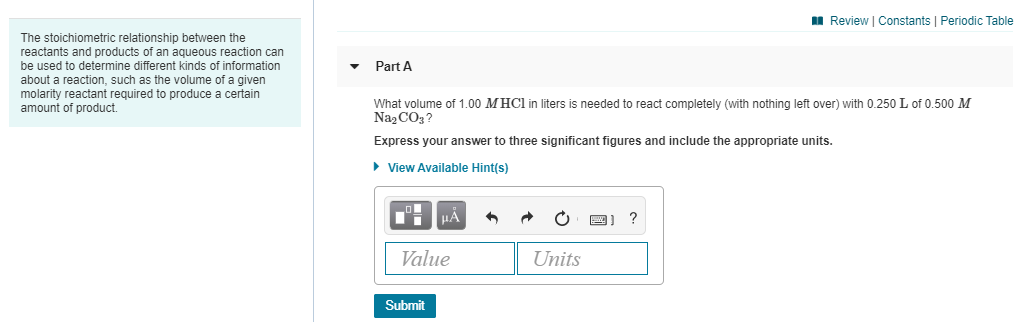Part A What volume of 1.00 M HCl in liters is needed to react completely (with nothing left over) with 0.250 L of 0.500 M Na2CO3? Express your answer to three significant figures and include the appropriate units. Part B A 419-mL sample of unknown HCl solution reacts completely with Na2CO3 to form 12.1 g CO2. What was the concentration of the HCl solution? Express your answer to three significant figures and include the appropriate units. A Review Constants Periodic...

• ### 9 of 28 A Review Constants | Periodic Table 2HCl(aq) + Na2CO3(aq) +2NaCl(aq) + H2O(l) +...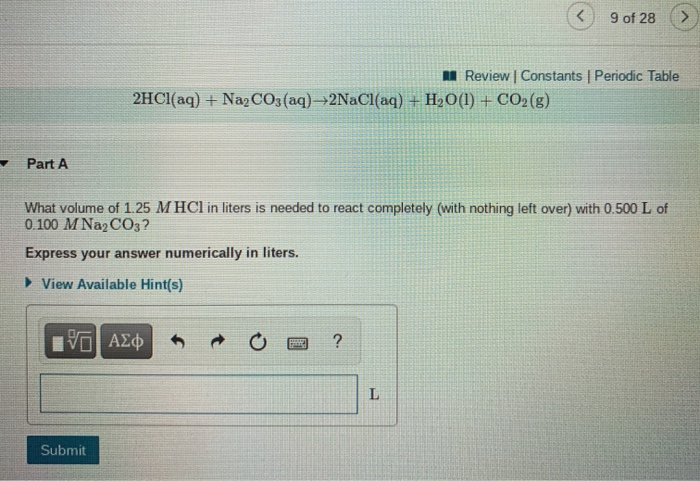9 of 28 A Review Constants | Periodic Table 2HCl(aq) + Na2CO3(aq) +2NaCl(aq) + H2O(l) + CO2(g) Part A What volume of 1.25 M HCl in liters is needed to react completely (with nothing left over) with 0.500 L of 0.100 M Na2CO3? Express your answer numerically in liters. ► View Available Hint(s) ΟΙ ΑΣΦ ? L Submit 9 of 28 Review | Constants Periodic Table Part B A 381-mL sample of unknown HCl solution reacts completely with Na2CO3 to...

• ### Metal carbonates react with acid to produce a salt, water, and carbon dioxide gas as seen...

Metal carbonates react with acid to produce a salt, water, and carbon dioxide gas as seen in the reaction below. Na2CO3(s)+2HCl(aq)→2NaCl(aq)+CO2(g)+H2O(l) If 1.45 g of Na2CO3 reacts with an excess amount of HCl, what volume of CO2 will be produced at STP?

• ### Calcium carbonate (CaCO3) reacts with stomach acid (HCl, hydrochloric acid) according to the following equation: CaCO3(s)...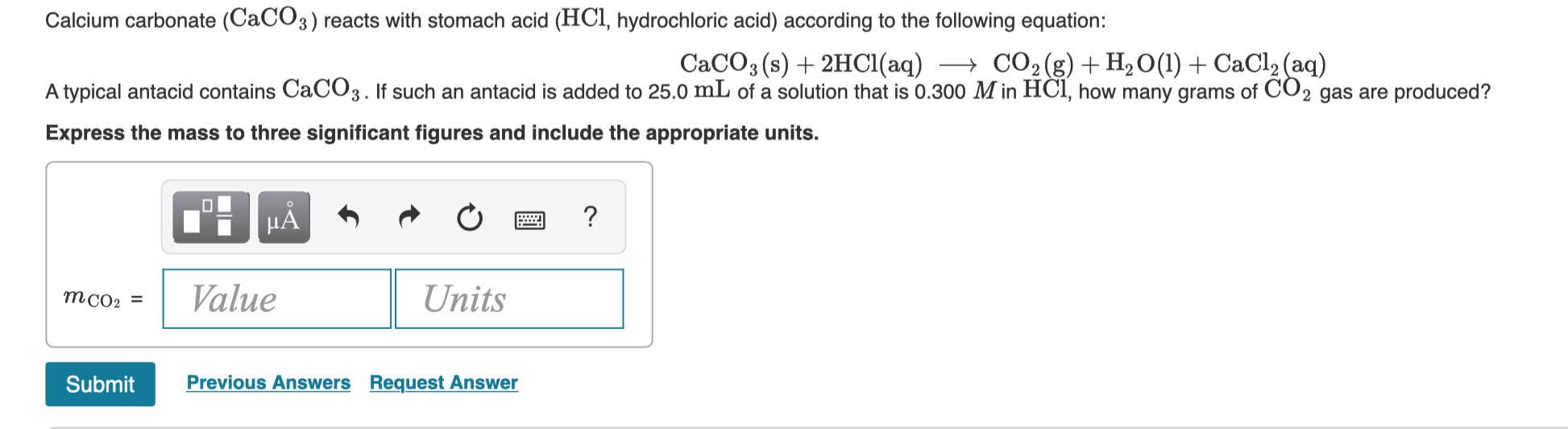Calcium carbonate (CaCO3) reacts with stomach acid (HCl, hydrochloric acid) according to the following equation: CaCO3(s) + 2HCl(aq) + CO2(g) + H2O(l) + CaCl2(aq) A typical antacid contains CaCO3. If such an antacid is added to 25.0 mL of a solution that is 0.300 M in HCl, how many grams of CO2 gas are produced? Express the mass to three significant figures and include the appropriate units. TI MÃ + + + a ? Value Units MCO: = Submit Previous...

• ### 1. What is the final volume in milliliters when 0.653 L of a 45.4 % (m/v)...

1. What is the final volume in milliliters when 0.653 L of a 45.4 % (m/v) solution is diluted to 24.0 % (m/v)? Express your answer with the appropriate units. 2. A 751 mL NaCl solution is diluted to a volume of 1.06 L and a concentration of 8.00 M . What was the initial concentration? Express your answer with the appropriate units. 3. What volume of 1.00 M HCl in liters is needed to react completely (with nothing left...

• ### 5.119 A 24.9-mL volume of hydrochloric acid reacts com pletely with 55.0 mL of aqueous Na2CO3....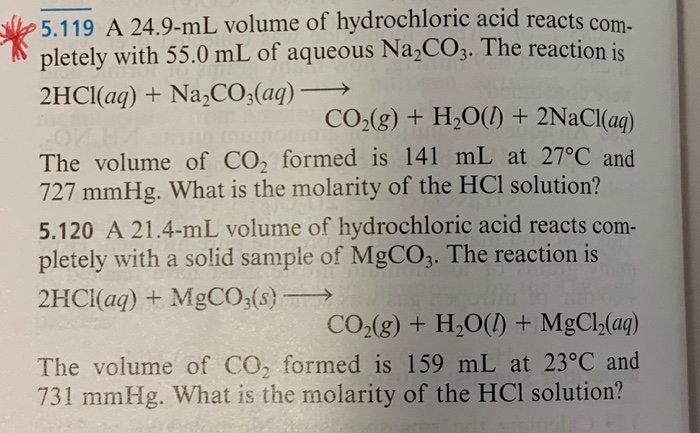5.119 A 24.9-mL volume of hydrochloric acid reacts com pletely with 55.0 mL of aqueous Na2CO3. The reaction is 2HCI(aq) NaCO3(aq) CO2(g) + H20() + 2NaCl(ag) The volume of CO, formed is 141 mL at 27°C and 727 mmHg. What is the molarity of the HCl solution? 5.120 A 21.4-mL volume of hydrochloric acid reacts com- pletely with a solid sample of MgCO3. The reaction is 2HCI(aq)MgCO3(s) CO2(g) + H2O() + MgCl (ag) The volume of CO, formed is 159...